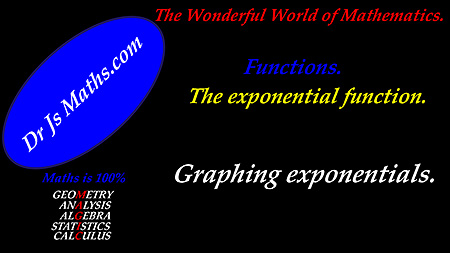Dr. J's Maths.com
Where the techniques of Maths
are explained in simple terms.

Functions - Exponential - main page.

The exponential function is important in explaining many events - especially those related to increasing or decreasing trends. It has the special characteristic that:

the rate at which the attribute being measured changes

is directly proportional to

the amount of that attribute at any given time.

Exponential equations are used in many situations - including finance (especially compound interest), growth of populations and radio-active decay.

The actual function was first considered in the context of a limiting value of a term by Jacob Bernoulli about 1683. In 1697, his brother Johann investigated the calculus of the exponential function. Leonhard Euler also joined the investigation at a later date (Johann had been his teacher).

The resources which can be accessed directly are:

 1.The Amazing number e. 2. Graphing exponential functions. 3. Differentiation approaches to exponential functions. 4. Integration of exponential functions. 5. Application of exponential integration to equations. 6. Area questions. 7. Natural growth and decay - applications. 8. Reverse Chain Rule questions. 9. Differentiate ... hence find questions. 10. Integration by substitution (non-RCR).

 Learning area Resource Basic indices Main page for indices for revision. The Amazing number e - Euler's number. Manipulating and graphing exponential functions.Manipulating and graphing exponential functions -Test Yourself 1. Manipulating and graphing exponential functions - Test Yourself 1 - Solutions. Differentiation of the exponential function.Exponential basic differentiation - Test Yourself 1. Exponential basic differentiation - Test Yourself 1 - Solutions. Exponential basic differentiation - Test Yourself 2. Exponential basic differentiation - Test Yourself 2 - Solutions. Applications of differerentiating exponentials. Exponential differentiation applications (max/min, etc) - Test Yourself 1. Exponential differentiation applications (max/min, etc) - Test Yourself 1 - Solutions. Multifunction differentiation with exponentials. Multifunction differentiation - Test Yourself 1. Multifunction differentiation - Test Yourself 1 - Solutions. Integration of the exponential function.Basic questions. Exponential basic Integration - Test Yourself 1. Exponential basic Integration - Test Yourself 1 - Solutions. Areas. Exponential - integration - calculating areas - Test Yourself 1. Exponential - integration - calculating areas - Test Yourself 1 - Solutions. Exponential - integration - calculating areas - Test Yourself 2. Exponential - integration - calculating areas - Test Yourself 2 - Solutions. Natural growth and decay. Natural Growth & decay - Test Yourself 1. Natural Growth & decay - Test Yourself 1 - Solutions. Growth and decay - Test Yourself 2. Growth and decay - Test Yourself 2 - Solutions. Modified natural growth and decay. (extension courses). Multifunction integration. Multifunction integration - Test Yourself 1. Multifunction integration - Test Yourself 1 - Solutions. Reverse Chain Rule. Integration - RCR - Test Yourself 1. Integration - RCR - Test Yourself 1 - Solutions. Differentiate ... hence find questions. Differentiate - Hence find - Test Yourself 1. Differentiate -Hence find - Test Yourself 1 - Solutions.

The basic strategies for differentiation are required for the exponential function also.

These strategies (including the Chain Rule, the Product Rule and the Quotient Rule) can be reviewed through the main differentiation page.